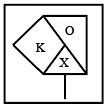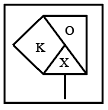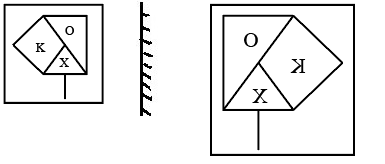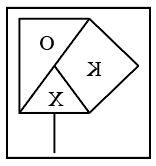Question 4

# Which of the option figures is the exact mirror image of the given problem figure when the mirror is held to the right side of the problem figure?Solution

Given figurethe above figure mirror image is given below held to the right side of the problem figurethen mirror image istherefore Option (B) Ans